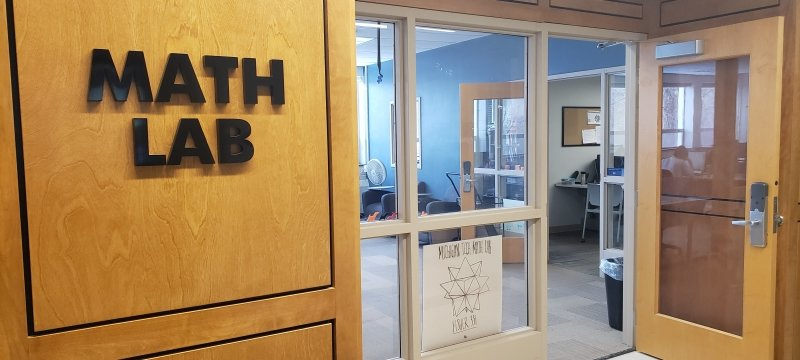Mathematical SciencesAlso in this section

# MathLab## Mission Statement

The Michigan Tech Math Lab shall enhance the understanding of core calculus concepts through the use of the Wolfram Mathematica program, as well as promoting an understanding of applied mathematics and general programming knowledge.

## General Lab Resources

The Math Lab Canvas page contains resources for:

• learning the basics of Mathematica
• learning Mathematica concepts, for other courses such as Differential Equations and Linear Algebra
• reserving the Lab's publicly available resources, such as 3D printers, Whiteboards, and Raspberry Pis
• assistance with calculus lab assignments
• general lab information

## Calculus Students

All Calculus Students are automatically enrolled into the Mathematica Lab course. The course consists of weekly assignments involving the use of the Wolfram Mathematica software. These assignments are worth ten percent of your overall grade for Calculus I, II, and III each. Assignment details and grading policies can be found on your lab course's Canvas page.

Students looking for help with their Mathematica Lab assignments may:

• Ask for help from a consultant in-person at the Math Lab
• Ask for help using Google Hangouts (Search for MTU Math Lab)